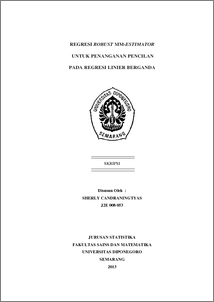# REGRESI ROBUST MM-ESTIMATOR UNTUK PENANGANAN PENCILAN PADA REGRESI LINIER BERGANDA

CANDRANINGTYAS, SHERLY (2013) REGRESI ROBUST MM-ESTIMATOR UNTUK PENANGANAN PENCILAN PADA REGRESI LINIER BERGANDA. Undergraduate thesis, UNIVERSITAS DIPONEGORO.Preview
PDF
398Kb

## Abstract

The multiple linear regression model is used to study the relationship between a dependent variable and more than one independent variables. Estimation method which is the most frequently be used to analyze regression is Ordinary Least Squares (OLS). OLS for linear regression models is known to be very sensitive to outliers. Robust regression is an important method for analyzing data contaminated by outliers. This paper will discuss the robust regression MM-estimator. This estimation is a combined estimation method which has a high breakdown value (LTS-estimator or S-estimator) and M-estimator. Generally, there are three steps for MM-estimator: estimation of regression parameters initial using LTS-estimators, residual and robust scale using M-estimator, and the final estimation parameter using M-estimator. The purpose of writing this paper are to detect outliers using DFFITS and determine the multiple linear regression equations containing outliers using robust regression MM-estimator. The data used is the generated data from software Minitab 14.0. Based on the analysis results can be concluded that data 21st, 27th, 34th are outliers and equation of multiple linear regression using robust regression MM-estimators is y ̂=3.97+0.392 x_1-0.810 x_2-0.263 x_3+ 0.968 x_4. Keywords : Multiple Linear Regression, Ordinary Least Square (OLS), Outliers, Robust Regression LTS-Estimator, Robust Regression M-Estimator, Robust Regression MM-Estimator

Item Type: Thesis (Undergraduate) H Social Sciences > HA Statistics Faculty of Science and Mathematics > Department of Statistics 42586 Mr Hasbi Yasin 03 Mar 2014 16:37 03 Mar 2014 16:37

Repository Staff Only: item control page